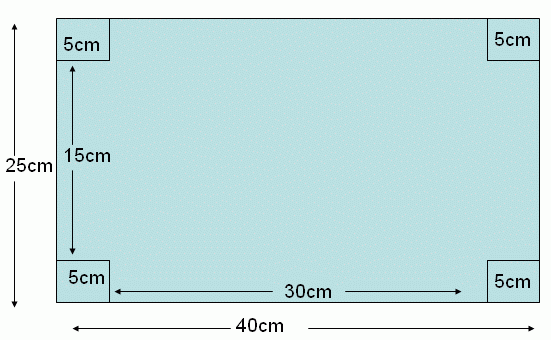Name: ___________________Date:___________________

kwizNET Subscribers, please login to turn off the Ads!
 Email us to get an instant 20% discount on highly effective K-12 Math & English kwizNET Programs!

### Grade 7 - Mathematics9.60 Word Problems on Cuboid - I

 Example: The length and width of a rectangular metal sheet are 40 cm and 25 cm respectively. Four squares each of side 5 cm are cut and removed from four corners of the sheet, and the remainder is folded to form a cuboid(without the top face). Find the volume. Solution:In the given rectangular sheet has length and breadth equal to 40 cm and 25 cm respectively. If four corner squares of the side 5 cm are removed and rest is folded into a cuboid. Then the length and width of the cuboid is reduced by twice the side of square. Therefore the length of the cuboid = 40 - (2 * 5) = 40 - 10 length (l)= 30 cm. The width of the cuboid = 25 - (2 * 5) = 25 - 10 width (b) = 15 cm. The height of the cuboid itself is equal to the side of the square removed. Therefore the height of the cuboid = 5 cm. Hence the volume of the cuboid is V = l * b * h V = 30 * 15 * 5 Volume = 2250 cubic centimeters. Directions: Read the above example carefully and answer the following questions. Also make 10 different examples of your own and illustrate each by making cuboids.Name: ___________________Date:___________________

### Grade 7 - Mathematics9.60 Word Problems on Cuboid - I

 Q 1: The length and width of a rectangular metal sheet are 30 cm & 25 cm respectively. Four squares each of side 5 cm are cut and removed from four corners of the sheet, and the remainder is folded to form a cuboid(without the top face). Find the volume.1400 cubic centimeters1600 cubic centimeters1500 cubic centimeters1800 cubic centimeters Q 2: The length and width of a rectangular metal sheet are 20 cm & 16 cm respectively. Four squares each of side 4.5 cm are cut and removed from four corners of the sheet, and the remainder is folded to form a cuboid(without the top face). Find the volume.348.5 cubic centimeters346.5 cubic centimeters345.5 cubic centimeters346.5 cubic centimeters Q 3: The width of a rectangular metal sheet is 9 cm. Four squares each of side 2 cm. are cut and removed from four corners of the sheet, and the remainder is folded to form a cuboid(without the top face). Find the length of the rectangular metal sheet if its volume is given by 90 cubic centimeters.18 centimeters11 centimeters19 centimeters13 centimeters Q 4: The length of a rectangular metal sheet is 35 cm. Four squares each of side 5 cm are cut and removed from four corners of the sheet, and the remainder is folded to form a cuboid(without the top face). Find the width of the rectangular sheet if its volume is given by 2500 cubic centimeters.26 centimeters24 centimeters30 centimeters35 centimeters Q 5: The width of a rectangular metal sheet is 40 cm. Four squares each of side 6 cm are cut and removed from four corners of the sheet, and the remainder is folded to form a cuboid(without the top face). Find the length of the rectangular sheet if its volume is given by 5544 cubic centimeters.54 centimeters43 centimeters45 centimeters44 centimeters Q 6: The length of a rectangular metal sheet are 25 cm. Four squares each of side 4.5 cm are cut and removed from four corners of the sheet, and the remainder is folded to form a cuboid(without the top face). Find the width of the rectangular metal sheet if its volume is given by 792 cubic centimeters.32 centimeters30 centimeters20 centimeters25 centimeters Question 7: This question is available to subscribers only! Question 8: This question is available to subscribers only!

#### Subscription to kwizNET Learning System costs less than \$1 per month & offers the following benefits:

• Unrestricted access to grade appropriate lessons, quizzes, & printable worksheets
• Instant scoring of online quizzes
• Progress tracking and award certificates to keep your student motivated
• Unlimited practice with auto-generated 'WIZ MATH' quizzes
• Child-friendly website with no advertisements

© 2003-2007 kwizNET Learning System LLC. All rights reserved. This material may not be reproduced, displayed, modified or distributed without the express prior written permission of the copyright holder. For permission, contact info@kwizNET.com
For unlimited printable worksheets & more, go to http://www.kwizNET.com.